# dc coupled non-inverting op-amp biased with split resistor biasing method

Inputs:
V
V

Results: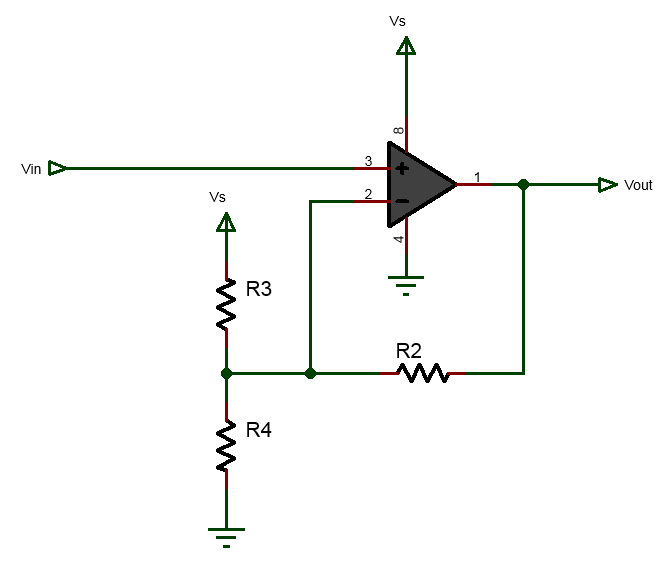## dc coupled non-inverting Op-Amp biased with VDB

This calculators helps you to bias an dc coupled inverting op-amp using single supply voltage.

### Equations Used:

$$A_v=-\frac{R_2}{R_1}$$
$$V_b =$$

# ac coupled non-inverting Op-Amp biased with split resistor

Inputs:
V
V

Results: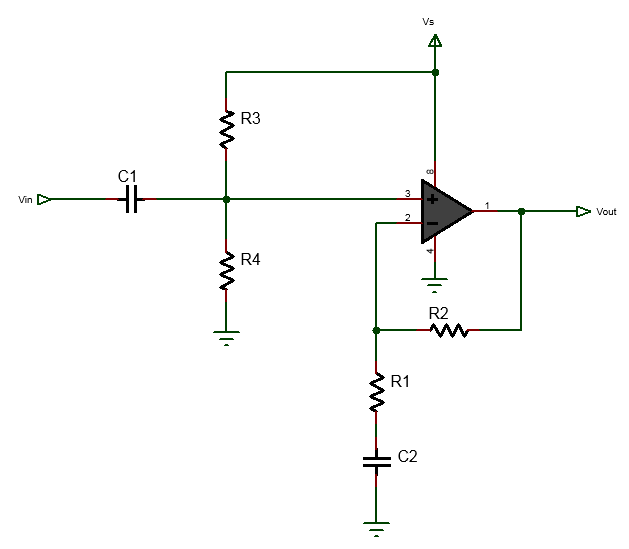## ac coupled non-inverting Op-Amp biased with split resistor

This calculator can be used to bias ac coupled inverting op-amp using the split resistor biasing methods.

### Equations Used:

$$C=\frac{F_{osc}}{2 N F_{w}}-1$$
where, Fosc is the frequency of the CPU, Fw is the output square wave frequency and N is the pre-scalar value.

# ac coupled non-inverting Op-Amp biased with VDB

Inputs:
V

V

Results: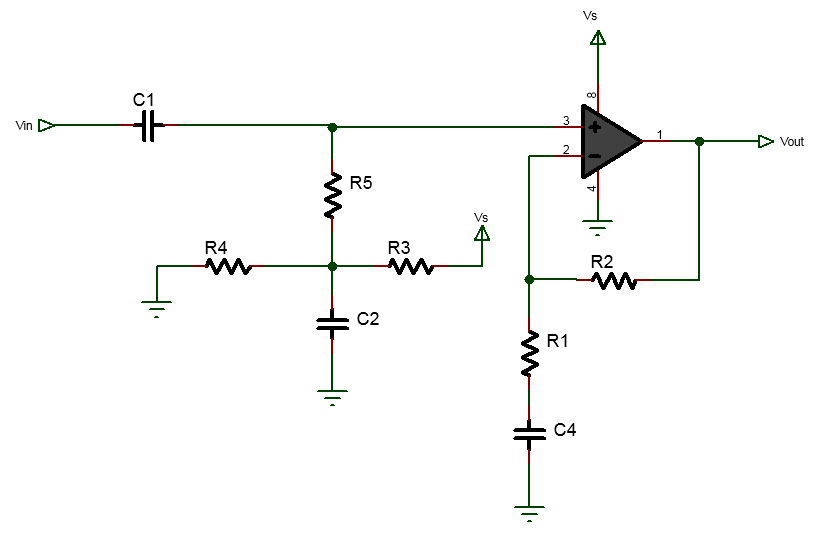## ac coupled non-inverting Op-Amp biased with VDB

The above calculator can be used to bias ac coupled inverting op-amp using voltage divider biasing method.

Design Example:

The following shows op-amp biased at 2.5V, cutoff frequency of 1KHz and with gain of 10.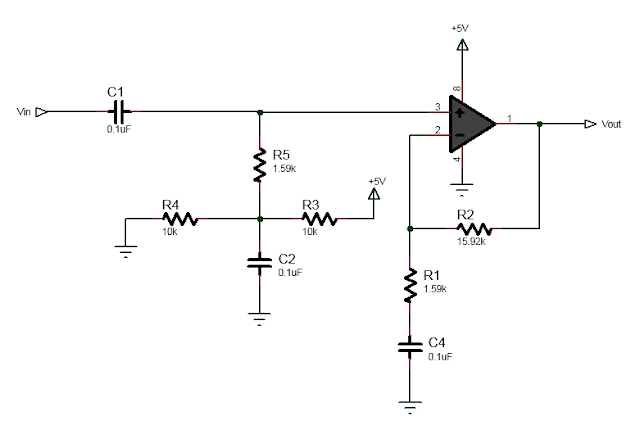# ac coupled non-inverting Op-Amp biased with voltage follower biasing

Inputs:
V

V

Results: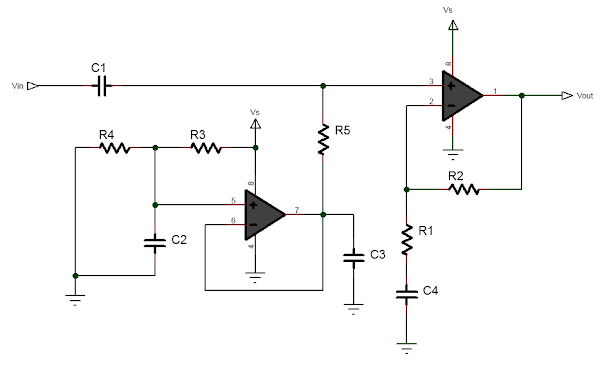## ac coupled non-inverting Op-Amp biased with voltage follower biasing

This calculator can be used to bias ac coupled inverting op-amp using the voltage follower biasing method. This biasing is same as voltage divider biasing but uses op-amp or transistor to buffer the bias voltage. The disadvatange of this biasing method is that, more circuit component are required. And the advantage is that for dc coupled op-amp amplifier, it reduces the resistor size and therefore less power consumption happens resulting in gain improvement and offset. Also it provides stable bias voltage. It should be used when there is extra op-amp available in the circuit and accurate and stable design are required.

Design example

Following is circuit design example:

The input and output signal waveform is shown below.

The frequency response graph is shown below.

### Equations Used:

$$A_v=1+\frac{R_2}{R_1}$$
$$V_b = \frac{R_4}{R_3+R_4}V_s$$
$$f_c = \frac{1}{2 \pi R_5 C_1} = \frac{1}{2 \pi R_1 C_4}$$